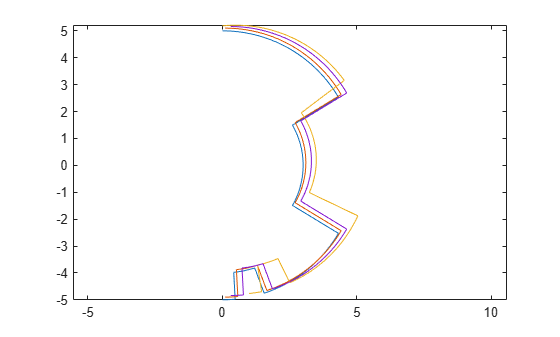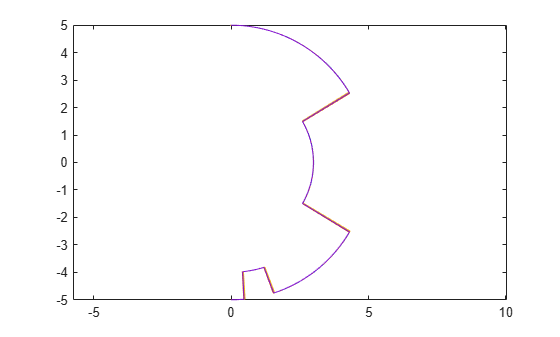# Compose a Series of Laser Scans with Pose Changes

Use the `matchScans` function to compute the pose difference between a series of laser scans. Compose the relative poses by using a defined `composePoses` function to get a transformation to the initial frame. Then, transform all laser scans into the initial frame using these composed poses.

Specify the original laser scan and offsets to generate a series of shifted laser scans. Iterate through the scans and transform the original scan based on each offset. Plot the laser scans to see the shifted data.

```ranges = zeros(300,4); angles = zeros(300,4); ranges(:,1) = 5*ones(300,1); ranges(11:30,1) = 4*ones(1,20); ranges(101:200,1) = 3*ones(1,100); angles(:,1) = linspace(-pi/2,pi/2,300); offset(1,:) = [0.1 0.1 0]; offset(2,:) = [0.4 0.1 0.1]; offset(3,:) = [-0.2 0 -0.1]; for i = 2:4 [ranges(:,i),angles(:,i)] = transformScan(ranges(:,i-1),angles(:,i-1),offset(i-1,:)); end [x,y] = pol2cart(angles,ranges); plot(x,y) axis equal```Perform scan matching on each laser scan set to get the relative pose between each scan. The outputs from the `matchScans` function are close to the specified offsets. The initial scan is in the initial frame, so the pose difference is `[0 0 0]`.

```relPoses(1,:) = [0 0 0]; for i = 2:4 relPoses(i,:) = matchScans(ranges(:,i),angles(:,i),... ranges(:,i-1),angles(:,i-1),'CellSize',1); end```

Use the `composePoses` function in a loop to get the absolute transformation for each laser scan. This function is defined at the end of the example. Transform each scan to get them all in the initial frame.

```transRanges = zeros(300,4); transAngles = zeros(300,4); transRanges(:,1) = ranges(:,1); transAngles(:,1) = angles(:,1); composedPoses(1,:) = [0 0 0]; for i = 2:4 composedPoses(i,:) = composePoses(relPoses(i,:),composedPoses(i-1,:)); [transRanges(:,i),transAngles(:,i)] = transformScan(ranges(:,i),angles(:,i),composedPoses(i,:)); end```

Plot the transformed ranges and angles. They overlap well, based on the calculated transformations from `matchScans`.

```[x,y] = pol2cart(transAngles,transRanges); plot(x,y) axis equal```Define the `composePoses` function. This function takes in the transformation of the initial frame to the base frame and the relative transformation from the initial frame to a second frame. For a series of laser scans, the `relative` input is the relative pose between the last two frames, and the `base` input is the composed pose over all previous scans.

You can also define this function in a separate script and save to the current folder.

```function composedPose = composePoses(relative,base) %Convert both poses (3-by-1 vector) to transformations (4-by-4 matrix) and multiply %together using pose2tform function. tform = pose2tform(base)*pose2tform(relative); % Extract translational vector and Euler angles as ZYX. trvec = tform2trvec(tform); eul = tform2eul(tform); % Concatenate the elements of the transform as [x y theta]. composedPose = [trvec(1:2) eul(1)]; % Function to convert pose to transform. function tform = pose2tform(pose) x = pose(1); y = pose(2); th = wrapTo2Pi(pose(3)); tform = trvec2tform([x y 0])*eul2tform([th 0 0]); end end```